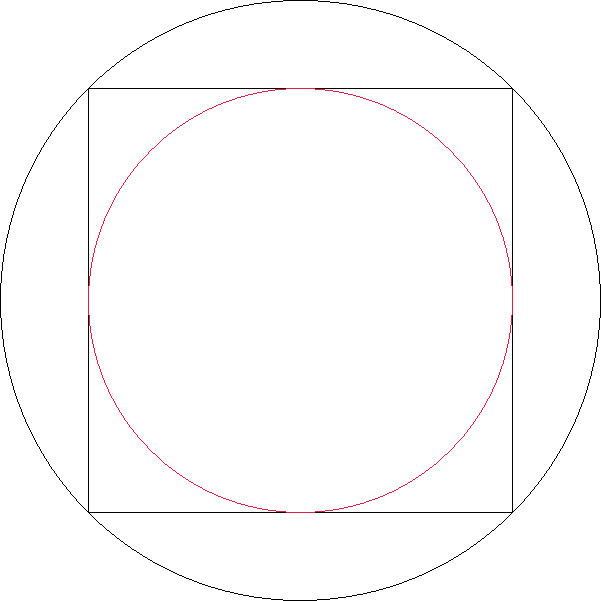Last week’s problem involved using your geometry/trigonometry skills to find areas of shapes. We start off with a large (black) circle which has a (black) square inscribed in it which in turn has a (red) circle inscribed in it:1. Let’s start off with the equation for the area of a circle: `A = π × r2` (where `r` is the radius)
2. Now let’s think about the equation for area of a square: `A = s2` (where `s` is the length of one of the sides)
3. Next we can say that the radius of the inner circle is `r1`.
4. After that let’s find the area of the red inner circle relative to `r1`: `A1 = π × r12`
5. Now let’s find the length of the diagonal of the square (`d`) in which the red circle is inscribed relative to `r1`. This will also be the diameter of the outer circle
1. `s = r1 + r1 = 2 × r1`
2. The above is true because the red circle is inscribed in the square.
3. `d2 = s2 + s2`
4. `d2 = 2 × s2`
5. `d = (2 × s2)1/2`
6. `d = s × 21/2`
7. `d = 2 × r1 × 21/2`
6. Next we should find the area of the black circle (`A2`), in which the black square is inscribed, relative to `r1`.
1. We will use `r2` to represent the radius of the outer circle:
1. `r2 = d / 2`
2. `r2 = (2 × r1 × 21/2) / 2`
3. `r2 = r1 × 21/2`
2. `A2 = π × r22`
3. `A2 = π × (r1 × 21/2)2`
4. `A2 = π × r12 × 2`
7. Lastly we should find the area of the black outer circle while excluding the area covered by the red inner circle:
1. Let’s make the area of the black doughnut shape be represented by `A3`.
2. `A3 = A2 - A1`
3. `A3 = (π × r12 × 2) - (π × r12)`
4. `A3 = (π × r12) + (π × r12) - (π × r12)`
5. `A3 = π × r12`

So after doing all of the math by using a little geometry and a little algebra we end up with the `A1` (red area) being equal to `A3` (black area).Even though the image makes it look like there is more red than black, there really isn’t. Interesting stuff, huh? 😎

### 1 Comment

#### POW – Circle, Square, Circle | Chris West's Blog · October 13, 2014 at 12:20 PM

[…] to this problem of the week became available a week after the POW was published and can be found here. […]